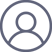柳渝《易经》学习 - 八卦的次序
2022-6-24 21:46A : 阳爻, B : 阴爻

(A+B)^0 = 1;

(A+B)^1 = A+B;

(A+B)^2 = A^2+ 2AB + B^2;

(A+B)^3 = A^3+ 3A^2B + 3AB^2+B^3;